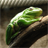# raster extraction

1765
3
02-25-2016 02:21 PMNew Contributor

I have a original tif file which has values from 2 to 28. From that original tif file, I am extracting cells having value >=10 using the following code. The code is working. However, when I open the new extracted tif file in GIS, I found that the cell values range from 10 30 instead of 10 to 28. I would really appreciate any help/ suggestion!

The code is provided below:

import arcpy, os

from arcpy import env

from arcpy.sa import *

#To overwrite output

arcpy.env.overwriteOutput = True

#Set environment settings

env.workspace = "C:/Subhasis/Highland/Project/Python_data"

outws="C:/Subhasis/Highland/Project/Python_data/HSA_10"

#checkout ArcGIS spatial analyst extension license

arcpy.CheckOutExtension("Spatial")

inraster = arcpy.ListRasters("*", "TIF")

for i in inraster:

flds = ("VALUE", "COUNT")

dct = {row:row for row in arcpy.da.SearchCursor(i, flds)}

sumcnt = sum(dct.values())

dct1 = {k:v for (k,v) in dct.items() if k >= 10}

sumcnt1 = sum(dct1.values())

percentage=(float(sumcnt1)/float(sumcnt))

print i,percentage

newraster = ExtractByAttributes(str(i), "VALUE>=10")

outname=os.path.join(outws,str(i))

newraster.save(outname)

1 Solution

Accepted SolutionsbyEsri Regular Contributor

for testing purposes I'd include (see highlighted 3 lines)

for i in inraster:

flds = ("VALUE", "COUNT")

dct = {row:row for row in arcpy.da.SearchCursor(i, flds)}

sumcnt = sum(dct.values())

dct1 = {k:v for (k,v) in dct.items() if k >= 10}

sumcnt1 = sum(dct1.values())

percentage=(float(sumcnt1)/float(sumcnt))

print i,percentage

`  arcpy.CalculateStatistics_management(str(i))`

`  maxValue = arcpy.GetRasterProperties_management(str(i), "MAXIMUM")`

print maxValue

newraster = ExtractByAttributes(str(i), "VALUE>=10")

outname=os.path.join(outws,str(i))

newraster.save(outname)

and the same for newraster

3 RepliesbyMVP Honored Contributor

Is the 30 actually in the data, or just listed as the upper limit in the symbology?New Contributor

No, 30 is not in the data. The upper limit is 28.byEsri Regular Contributor

for testing purposes I'd include (see highlighted 3 lines)

for i in inraster:

flds = ("VALUE", "COUNT")

dct = {row:row for row in arcpy.da.SearchCursor(i, flds)}

sumcnt = sum(dct.values())

dct1 = {k:v for (k,v) in dct.items() if k >= 10}

sumcnt1 = sum(dct1.values())

percentage=(float(sumcnt1)/float(sumcnt))

print i,percentage

`  arcpy.CalculateStatistics_management(str(i))`

`  maxValue = arcpy.GetRasterProperties_management(str(i), "MAXIMUM")`

print maxValue

newraster = ExtractByAttributes(str(i), "VALUE>=10")

outname=os.path.join(outws,str(i))

newraster.save(outname)

and the same for newraster